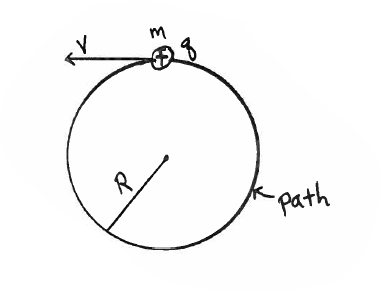# Problem: A small sphere with positive charge q = 5.00 x 10 -4 C and mass 2.00 x 10 -5 kg is moving in a region of uniform magnetic field. The object moves counterclockwise in a path that is a horizontal circle with radius R = 0.0080 m, as shown in the sketch. The speed of the sphere is a constant 3.0 m/s. Gravity can be neglected. What are the magnitude and direction of the magnetic field in the region? Write the direction in blank provided and also show the direction in the sketch. (Note: The acceleration for uniform circular motion is arad = v2/R.) Ans. B =  direction =

###### FREE Expert Solution
84% (432 ratings)
###### Problem Details

A small sphere with positive charge q = 5.00 x 10 -4 C and mass 2.00 x 10 -5 kg is moving in a region of uniform magnetic field. The object moves counterclockwise in a path that is a horizontal circle with radius R = 0.0080 m, as shown in the sketch. The speed of the sphere is a constant 3.0 m/s. Gravity can be neglected. What are the magnitude and direction of the magnetic field in the region? Write the direction in blank provided and also show the direction in the sketch. (Note: The acceleration for uniform circular motion is arad = v2/R.)

Ans. B =

direction =Frequently Asked Questions

What scientific concept do you need to know in order to solve this problem?

Our tutors have indicated that to solve this problem you will need to apply the Circular Motion of Charges in Magnetic Fields concept. You can view video lessons to learn Circular Motion of Charges in Magnetic Fields. Or if you need more Circular Motion of Charges in Magnetic Fields practice, you can also practice Circular Motion of Charges in Magnetic Fields practice problems.

How long does this problem take to solve?

Our expert Physics tutor, Juan took 3 minutes and 39 seconds to solve this problem. You can follow their steps in the video explanation above.